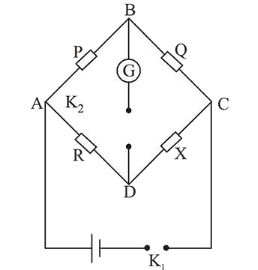# In a Wheatstone bridge (see fig.),

Question:

In a Wheatstone bridge (see fig.), Resistances $P$ and $Q$ are approximately equal. When $\mathrm{R}=400 \Omega$, the bridge is balanced. On interchanging $P$ and $Q$, the value of $R$, for balance, is $405 \Omega$. The value of $\mathrm{X}$ is close to:1. (1) $401.5 \mathrm{ohm}$

2. (2) $404.5 \mathrm{ohm}$

3. (3) $403.5 \mathrm{ohm}$

4. (4) $402.5 \mathrm{ohm}$

Correct Option: , 4

Solution:

(4)